# Quadrature formula of highest algebraic accuracy

A formula of the type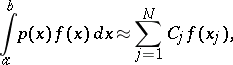(1)

where the weight functionis assumed to be non-negative on, where the integralsexist and where, moreover,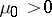. The nodes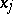of formula (1) are the roots of a polynomial of degreeorthogonal onwith the weight function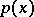, and the weights are defined by the condition that (1) be an interpolatory formula. A quadrature formula of this type has algebraic accuracy, i.e. it is exact for all algebraic polynomialsof degree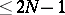and is not exact for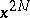; it is known as a quadrature formula of Gaussian type.

This concept can be generalized as follows. Consider the quadrature formula(2)

withnodes, where the nodes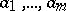are pre-assigned (fixed) whileare so chosen that (2) be a quadrature formula of highest algebraic accuracy. LetFormula (2) is exact for all polynomials of degreeif and only if it is an interpolatory quadrature formula and the polynomial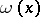is orthogonal onwith the weight function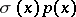to all polynomials of degree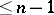. This reduces the question of the existence of a quadrature formula that is exact for all polynomials of degree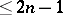to the problem of determining a polynomialof degreethat is orthogonal onwith the weight function, and to examining the properties of its roots. If the roots ofare real, simple, lie in, and none of them is one of the fixed nodes, the required quadrature formula exists. If, moreover,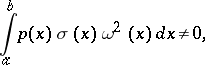then the algebraic accuracy of the formula is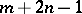.

Under the above assumptions concerning the weight function, a polynomialof degree, orthogonal onwith the weight function, is defined uniquely (up to a non-zero constant factor) in the following special cases.

1),is arbitrary. The single fixed node is an end-point of the interval, with the only condition that it be finite.

2)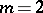,is arbitrary. The two fixed nodes are the end-points of the interval, provided they are finite.

3)is arbitrary,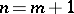. The fixed nodes are the roots of a polynomial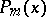that is orthogonal onwith the weight function.

In cases 1) and 2), the polynomialis orthogonal relative to the weight function, which is of fixed sign on, and therefore its roots are real, simple, lie inside, and are consequently distinct from. The quadrature formula (2) exists, its coefficients are positive and its algebraic accuracy is. Quadrature formulas corresponding to the cases 1) and 2) are called Markov formulas.

In case 3), the weight functionchanges sign onand this complicates the inspection of the roots of. Ifand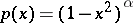, where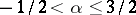, then the roots oflie insideand separate the roots of: Between any two consecutive roots ofthere is exactly one root of(see ). With this weight function, the quadrature formula (2) exists and is exact for all polynomials of degree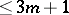; however, one cannot state that its algebraic accuracy is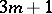. For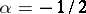and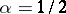the nodes and the coefficients of the quadrature formula can be specified explicitly (see ); the algebraic accuracy in the former case is increased to, and in the second case to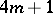. For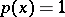and the interval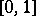, the nodes and the coefficients have been computed for the quadrature formula (2) (with fixed nodes of type 3)) for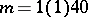(i.e.varying from 1 to 40 with step 1) (see ); the algebraic accuracy isifis even and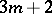ifis odd. A quadrature formula (2) with fixed nodes of type 3) also exists for the intervaland the weight function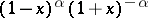for, and the nodes and the coefficients can be explicitly specified (see ).